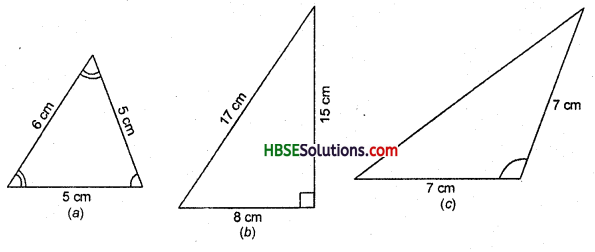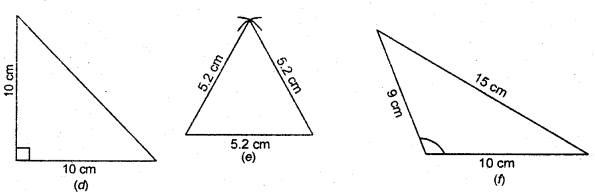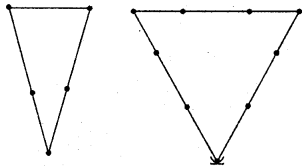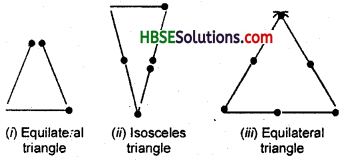# HBSE 6th Class Maths Solutions Chapter 5 Understanding Elementary Shapes Ex 5.6

Haryana State Board HBSE 6th Class Maths Solutions Chapter 5 Understanding Elementary Shapes Ex 5.6 Textbook Exercise Questions and Answers.

## Haryana Board 6th Class Maths Solutions Chapter 5 Understanding Elementary Shapes Exercise 5.6

Question 1.
Name the types of following triangles :
(a) Triangle with lengths of sides 7 cm, 8 cm and. 9 cm.
(b) ΔABC with AB = 8.7 cm, AC = 7 cm arid BC = 6 cm.
(c) ΔPQR such that PQ = QR = PR = 5 cm.
(d) ΔDEF with m ∠D = 90°.
(e) ΔXYZ with m∠Y = 90° and XY = YZ.
(f) ΔLMN with m ∠L = 30°, m ∠M = 70° and m ∠N = 80°.
Solution:
(a) Scalene triangle.
(b) Scalene triangle.
(c) Equilateral triangle.
(d) Right-angled triangle.
(e) Isosceles right-angled triangle.
(f) Acute-angled triangle.Question 2.
Match :

 Measures of Triangle Type of Triangle (i)      3 sides of equal length (ii)   2 sides of equal length (iii) All sides are of different length (iv)      3 acute angles (v)      1 right angle (vi)      1 obtuse angle (vii)      1 right angle with two sides of equal length (a)    Scalene (b)    Isosceles right-angled (c)    Obtuse-angled (d)     Right-angled (e)   Equilateral (f) Acute-angled (g) Isosceles

Solution:
(i) → (e),
(ii) →(g),
(iii) → (a),
(iv) → (f),
(v) → (d),
(vi) → (c),
(vii) → (b).

Question 3.
Name each of the following triangles in two different ways : (You may judge the nature of the angle by observation).Solution:
(a) Isosceles, Acute-angled.
(b) Scalene, Right-angled.
(c) Isosceles, Obtuse-angled.
(d) Isosceles, Right-angled.
(e) Equilateral, Acute-angled.
(f) Scalene, Obtuse-angled.Question 4.
Try to construct triangles using matchsticks. Some are shown here. Can you make a triangle with(a) 3 matchsticks ?
(b) 4 matchsticks ?
(c) 5 matchsticks ?
(d) 6 matchsticks ?
(Remember, you have to use all the available matchsticks in each case)
Name the type of triangle in each case.
Solution:
(a) Yes, we can make an equilateral triangle with 3 matchsticks. [Fig. (i)]
(b) No, we cannot make a triangle with 4 matchsticks.
(c) Yes, we can make an isosceles triangle with 5 matchsticks. [Fig. (ii)]
(d) Yes, we can make an equilateral triangle with 6 matchsticks. [Fig. (iii)]